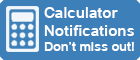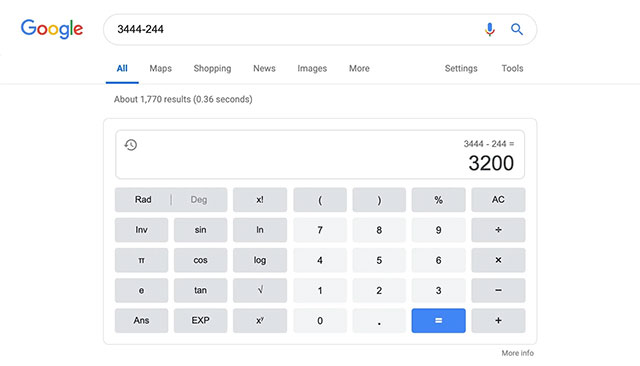Calculator Library
Free Online CalculatorsHome » 87 Calculators » Blog » The Google Calculator

Huge Domain Name Sale | Free Domains
Domain names on sale at crazy prices. Register a new domain for FREE when you buy another service.The Google Calculator is a powerful tool built into the Google search engine and allows users to perform a wide range of mathematical calculations quickly and easily. It was first introduced in July 2012 and has since become a popular tool for people looking to perform calculations without needing a dedicated calculator app or device.

This full-featured calculator can be accessed by typing a mathematical expression or equation into the Google search bar and hitting enter. The calculator will then provide the calculation result, along with a visual representation of the calculation process. Of course, it can handle basic arithmetic, but it also supports more advanced mathematical functions such as trigonometry, logarithms and statistical functions.

One of the key benefits of the Google Calculator is its simplicity and ease of use. Because it's integrated into the Google search engine, users don't need to navigate to a particular website or app to use it. This makes it an ideal tool for people who need to perform quick calculations on the go or don't have access to a dedicated calculator.

One of the standout features of the Google Calculator is its ability to understand and interpret natural language queries. This means that users can type out their calculations and conversions in a similar way to how they would say them out loud. For example, a user can type in "3+4", and the calculator will return the result "7". They can also type in more complex calculations such as "sin(45)" or "5 inches to cm" and the calculator will provide the correct result. Let's look at some of the other features of this calculator.

### Mathematical Calculations

The Google Calculator can perform basic arithmetic operations such as addition, subtraction, multiplication and division, square roots and percentages. However it also has some advanced capabilities that make it useful for more complex calculations. For example, it can be used to solve equations and find the roots of a function.

These features can be beneficial when working with advanced mathematical concepts such as algebra, trigonometry or calculus. This includes trigonometric functions such as sine, cosine and tangent, logical operators like AND, OR and NOT, and more advanced functions such as logarithms, inverse functions and factorials. When performing these calculations, it can handle parentheses and brackets which are often necessary when solving more complex equations.

Another essential feature of the Google Calculator is the ability to calculate statistical functions such as the mean, median and mode of a set of numbers. To solve these equations, you can just type them into the search bar, and the Google Calculator will provide you with the correct solution.

Many other diverse calculations can be performed with this calculator, such as working out the cost of a mortgage, the fuel efficiency of a vehicle or the area and circumference of common geometric shapes such as circles, squares and triangles.

### Built-In Functions

The Google Calculator has several built-in functions that can perform specialized calculations. For example, it has a mortgage calculator that can help users determine their monthly mortgage payments and a fuel consumption calculator that can help users determine how much fuel they need for a given distance. It also has several financial functions, such as the ability to calculate the present value of an annuity or the future value of an investment.

This calculator also includes a variety of commonly used mathematical constants such as pi, e and the square root of 2, which can be accessed by typing their names into the calculator.

### Multiple Calculations

The ability to handle multiple calculations at once is another valuable feature of the Google Calculator. Users can enter various equations separated by a semicolon, and the calculator will solve each one in turn and display the results. This is especially useful for users who need to perform multiple calculations as part of a larger project or for those working with a data set.

### Charts and Graphs

One of the coolest features of the Google Calculator is its ability to display the result of a calculation as a chart or graph. This can be particularly useful for visualizing data or understanding the relationship between different variables.

### Conversions

The Google Calculator also offers a wide range of other valuable features that make it more powerful than a traditional calculator. For example, it can handle conversions between different units of measurement, such as distance, weight, length, volume and temperature. Let's say you're converting between pounds and kilograms or miles and kilometers. You can simply type "200 pounds in kg" or "5 miles in km" into the search bar and it will provide you with the correct conversion. This can be especially helpful when working with measurements in science, engineering or cooking.

Users can also convert between different currencies using the latest exchange rates. Simply type in the amount you want to convert and the desired currency, and the calculator will give you the equivalent amount in the other currency.

### Usage History

The Google Calculator keeps a history of your calculations, allowing you to easily go back and refer to previous calculations or use them as the starting point for new calculations.

### Customization

Users can customize the calculator's display by changing the font size and color, as well as the background color. This can make it easier to read the calculator or make it more visually appealing.

### Definitions And Help

In addition to performing calculations and conversions, the Google Calculator provides information and definitions for various mathematical and scientific concepts. For example, if a user types "what is the law of gravity?" the calculator will provide a brief summary of the law and its importance in physics. There's also a built-in help feature to assist users unsure how to enter a particular calculation or conversion. They can simply type "help" into the calculator, which will provide them with a list of available operations and instructions on how to use them.

## Conclusion

The only real drawback of the Google Calculator is that it's not as powerful as some dedicated calculators or apps. It may not be able to handle highly complex calculations or provide as much detail as some other options. However, the Google Calculator is more than sufficient for most everyday calculations and conversions.

Overall, the Google Calculator is a valuable tool for anyone who needs to perform mathematical calculations quickly and easily. It's simple to use, powerful and versatile and can be accessed anywhere with an internet connection. It's also constantly being updated and improved, making it a reliable and valuable resource for users. Whether you're a student, a business professional or just someone who needs to do some quick math, the Google Calculator is a great tool to have at your disposal.

 Previous Post« World's Most Popular Calculators Next PostPercentage Calculators »